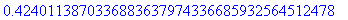Problem 8:

A square plate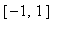xis at temperature.  At time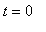the temperature is increased to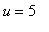along one of the four sides while being held at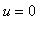along the other three sides, and heat then flows into the plate according to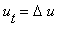.  When does the temperature reachat the center of the plate?

Solution:

This is a standard heat-equation problem with homogeneous boundary conditions.  It will be slightly more convenient to take the plate as [0,2] x [0,2], and the side withwill be taken as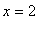.  The solution is obtained as the sum of a steady-state solution vwith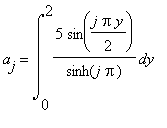so that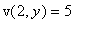, and a solution of the equation with homogeneous boundary conditions,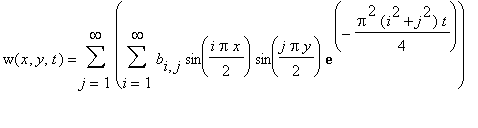with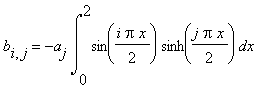so that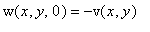.

 > aj:= int(5/sinh(j*Pi)*sin(j*Pi*y/2),y = 0 .. 2) assuming j::posint;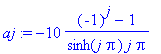> bij:= -aj*int(sin(i*Pi*x/2)*sinh(j*Pi*x/2),x = 0 .. 2) assuming i::posint,j::posint;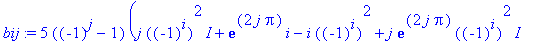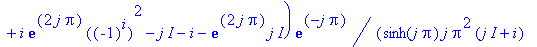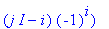> bij:= simplify(evalc(convert(%,exp))) assuming i::posint,j::posint;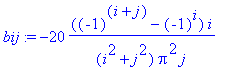We want to evaluate this at x=1, y=1.  By symmetry it is obvious that v(1,1) = 5/4.

As for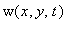, the factor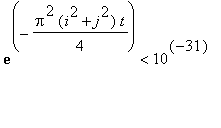for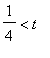(some preliminary investigation assured us that the answer we want should be somewhere between about 1/4 and 1) if.  So this approximation to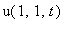should be accurate to approximately 30 decimals when: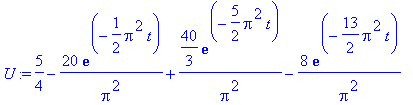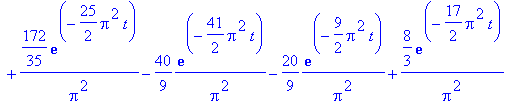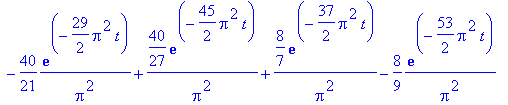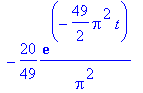Now to solve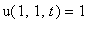: